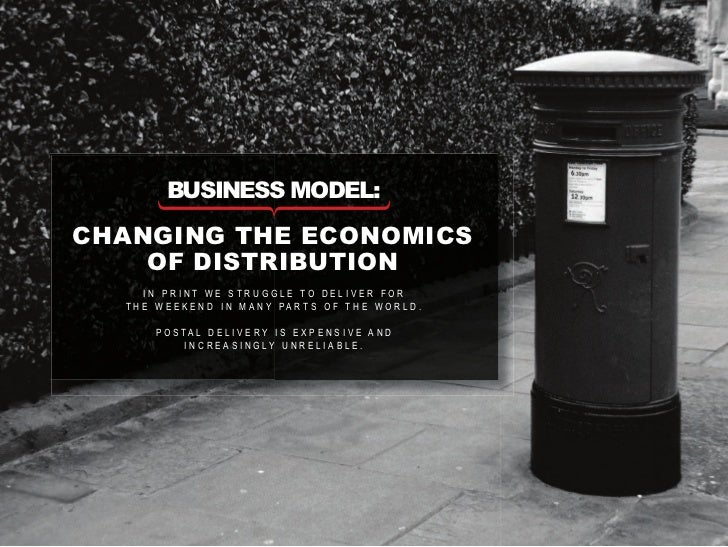Successfully reported this slideshow.Upcoming SlideShare
×

# marketing: the five p’s reimagined: Lean back media: the shock of the old

153,923 views

Published on

marketing: the five p’s reimagined: product: t h e d e m a n d f o r i n t e l l i g e n t, i m m e r s i v e l e a n b a c k i s a b s o l u t e b u t t h e p o t e n t i a l f o r l e a n - b a c k d i g i ta l to deliver an enhanced experience is still to be explored. price: r e a d e r r e l at i o n s h i p w i t h c o n t e n t b e i n g r e d e f i n e d and there are few price-point benchmarks. place: we can be everywhere, but how much power should we allow d i g i ta l s a l e s c h a n n e l s , s u c h a s i t u n e s a n d a m a z o n , t o e x e r t ? promotion:t h e pa r a d o x o f i n f i n i t e c h o i c e m e a n s s o c i a l i s a k e y d r i v e r and in an age of media consumption as a form of social e x p r e s s i o n , h o w d o w e s tay r e l e va n t a n d a s p i r at i o n a l ? people: m a s s i n t e l l i g e n c e i s a g l o b a l m a r k e t, but how do we serve them?

Published in: Technology, Education
• Full Name
Comment goes here.

Are you sure you want to Yes NoAre you sure you want to  Yes  No

Are you sure you want to  Yes  No